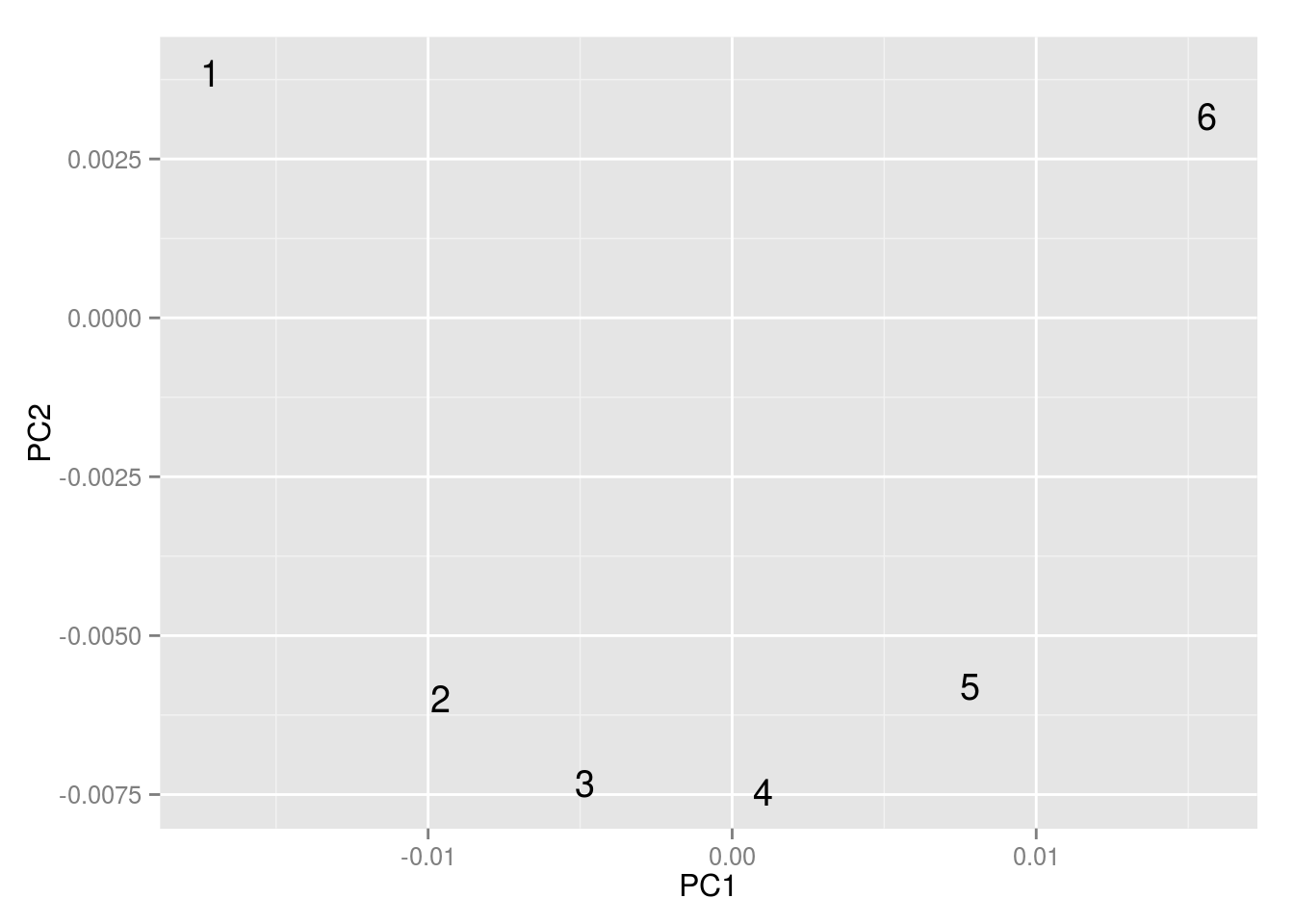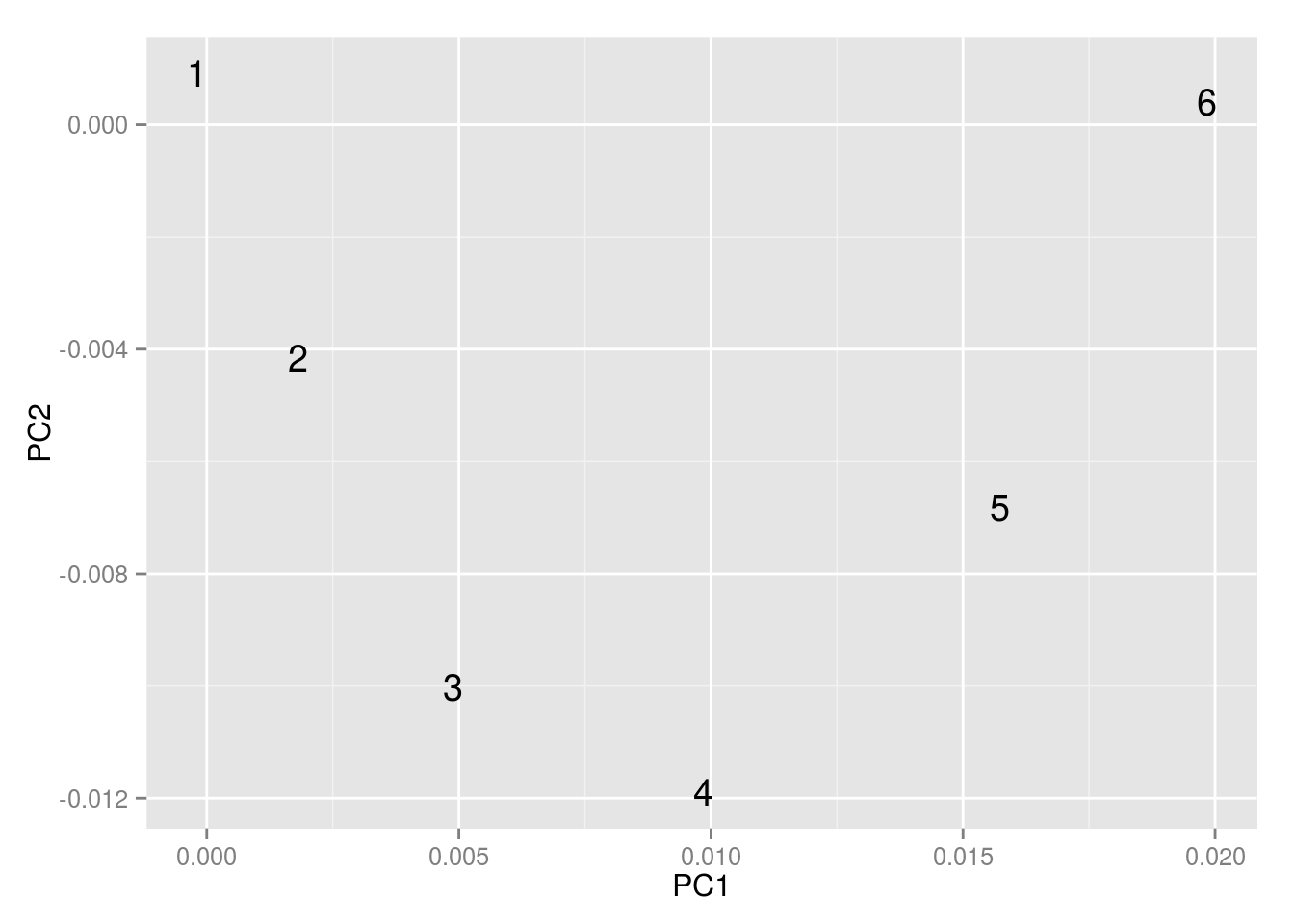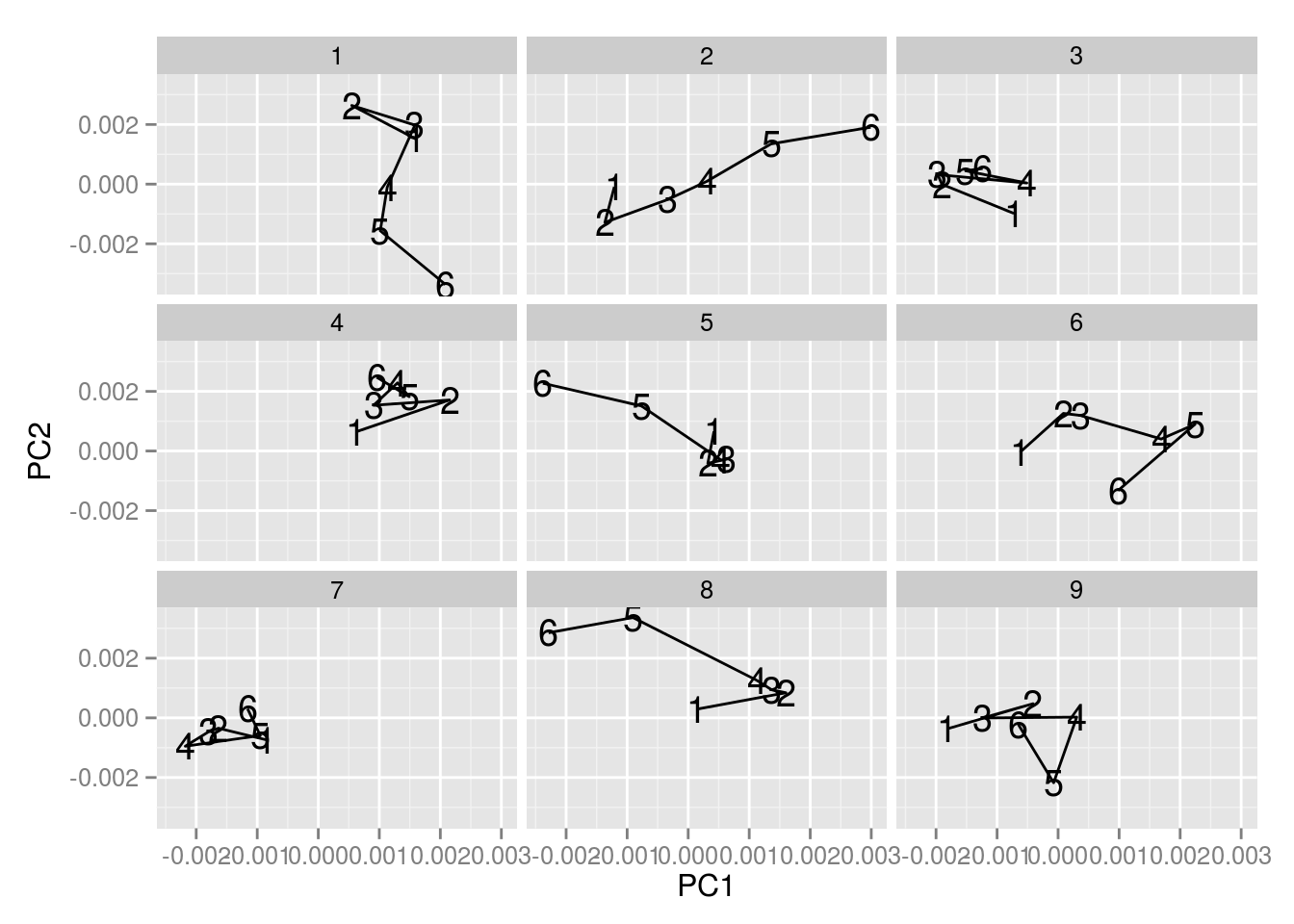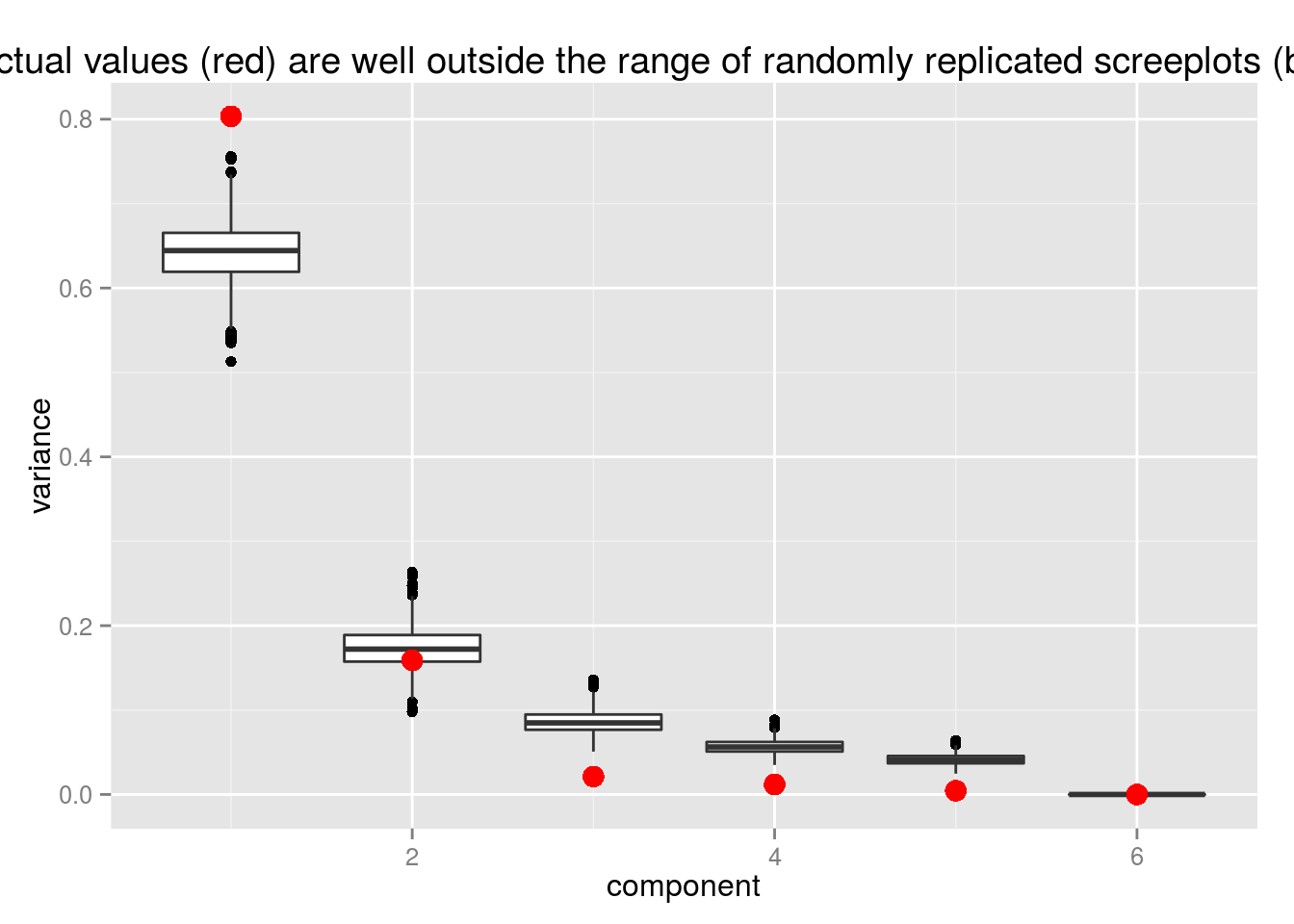A couple points about randomly generating PCA arcs.

First read in my data from the real thing.

library(dplyr)
##
## Attaching package: 'dplyr'
##
## The following object is masked from 'package:stats':
##
##     filter
##
## The following objects are masked from 'package:base':
##
##     intersect, setdiff, setequal, union
library(tidyr)
library(ggplot2)

#populates collective
load(url("http://benschmidt.org/arcInputs.RData"))

First, my Principal Components plot showing arcs.

masterPCAArcs = prcomp(collective)

a = collective %*% masterPCAArcs$rotation[,c(1,2)] %>% as.data.frame %>% mutate(doc=1:nrow(collective)) ggplot(as.data.frame(a)) + geom_text(aes(x=PC1,y=PC2,label=doc))What does a comparable random sample look like? The same length and standard deviation. It also gets arcs. averageChangesInTheSet = mean(apply(collective,2,sd)) randomData = function () { random = rnorm(nrow(collective)*ncol(collective),mean = 0,sd=averageChangesInTheSet) random = matrix(random,ncol=ncol(collective),nrow=nrow(collective)) #And then use cumsum so it's a walk, not just random data. random = apply(random,2,cumsum) randomPCAArcs = prcomp(random) a = random %*% randomPCAArcs$rotation %>% as.data.frame %>% mutate(doc=1:nrow(collective))
list(data = random,PCA=randomPCAArcs,plottable=a)
}

random = randomData()

ggplot(as.data.frame(random$plottable)) + geom_text(aes(x=PC1,y=PC2,label=doc))Out-of-sample data looks random-walk-y randomFrame = function(n) { data = randomData()$data
a = data %*% random$PCA$rotation %>% as.data.frame %>% mutate(doc=1:nrow(collective)) %>% as.data.frame
a$sample = n return(a) } random = lapply(1:9,randomFrame) %>% rbind_all ggplot(random,aes(x=PC1,y=PC2,label=doc)) + geom_text() + geom_path() + facet_wrap(~sample)# Scree Plots. This is the important one for me. Anything will turn up arcs, and this method is effectively a way of searching for them. But scree plots let us see whether those arcs are actually capturing most of the variance in the data. I run 1,000 random samples, and see how their scree plots compare to this one. The first component is more important than any of those thousand, and the third and fourth are less important than any of them. Variance (like in a scree plot) is very heavily concentrated in the first direction in the actual data (red–about 80% of variation is on the first component, and almost none is on the last 4.) I think there’s some question about whether the second dimension is random. variance = function(p) {p$sdev^2 / sum(p$sdev^2)} random = as.data.frame(t(replicate(1000,variance(randomData()$PCA)))) %>% tidyr::gather(component,variance,V1:V6,convert = T) %>% mutate(component = as.numeric(gsub("V","",component)))

ggplot(random,aes(x=component,y=variance,group=component)) + geom_boxplot() + geom_point(data=data.frame(variance = variance(masterPCAArcs),component=1:6),color="red",size=4) + labs(title="actual values (red) are well outside the range of randomly replicated screeplots (boxes)")